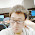### leetcode Question 110: Text Justification

Test Justification

Given an array of words and a length L, format the text such that each line has exactly L characters and is fully (left and right) justified.
You should pack your words in a greedy approach; that is, pack as many words as you can in each line. Pad extra spaces ' ' when necessary so that each line has exactly L characters.
Extra spaces between words should be distributed as evenly as possible. If the number of spaces on a line do not divide evenly between words, the empty slots on the left will be assigned more spaces than the slots on the right.
For the last line of text, it should be left justified and no extra space is inserted between words.
For example,
words["This", "is", "an", "example", "of", "text", "justification."]
L16.
Return the formatted lines as:
[
"This    is    an",
"example  of text",
"justification.  "
]

Note: Each word is guaranteed not to exceed L in length.
Corner Cases:
• A line other than the last line might contain only one word. What should you do in this case?
In this case, that line should be left-justified.

Analysis:

The idea of my solution is not efficient, but easily understood.
It is easier to follow the Python code below.

Step 1: Find the level number for each word. Assuming that there is at least 1 space between two words.
Step 2: Find the number of word in each level and the total spaces needed in that level.
Step 3: Compute the number of spaces M in each level that is needed between two words. If the spaces are not evenly distributed. Compute the number of words where 1+M spaces is needed, and the between the rest of words there needed M spaces.
Step 4: Write the levels which has only one word to the result.
Step 5: Find the levels that the last word that level ends with '.' (which is the sentence end).
Step 6: Write the levels to the result.
Step 7: Return result.

### Code(Python):

class Solution:
# @param words, a list of strings
# @param L, an integer
# @return a list of strings
def fullJustify(self, words, L):

lens = 0    # word length + single space in current level
result = [] # result list of strings
single_level = [] # list of strings in current level

for word in words:
lens += len(word)
if lens <= L:
single_level.append(word)   # add word into current level
lens += 1   # add single space length
else: #if word cannot be in current level

#get result string add to final results
result.append(self.proc_sinlge_lev(single_level, L, False))
#refresh the current level
single_level = []
single_level.append(word)
lens = len(word) + 1

#handle the last level of words
result.append(self.proc_sinlge_lev(single_level, L, True))
#return final result
return result

# @param single_level, a list of strings
# @param L, an integer
# @param last, an bool (True if current level is the last level)
def proc_sinlge_lev(self,single_level, L, last):

#last level no extra spaces inserted
if last == True:
if len(single_level) == 1:  #only 1 word in current level
str_lev = single_level.ljust(L)
else:
str_lev = ""  #result string
for str in single_level[0:-1]:
str_lev += str.ljust(len(str)+1) # 1 is a single space
str_lev += single_level[-1] # add the last word
str_lev = str_lev.ljust(L) # fill out spaces
return str_lev
else:
# compute lens of this level
if len(single_level) == 1: # only 1 word in current level
str_lev = single_level.ljust(L)
else:
lev_len = sum(len(s) for s in single_level) # sum of word length in current level
sp = L-lev_len  # total space numbers
first = sp % (len(single_level)-1)  # how many spaces needed when the spaces can not be evenly divided
sp_s = sp / (len(single_level)-1) # how many spaces are needed between two words
str_lev ="" # result string
for str in single_level[0:-1]:
if first != 0:  # add one more spaces from the left
str_lev += str.ljust(len(str)+sp_s+1)
first -=1
else:
str_lev += str.ljust(len(str)+sp_s)

str_lev += single_level[-1] #add last word

return str_lev



### Code(C++):

class Solution {
public:
vector<string> fullJustify(vector<string> &words, int L) {
// Start typing your C/C++ solution below
// DO NOT write int main() function
vector<string> res;
vector<int> lev;

int len=0;
int l=0;

for (int i=0;i<words.size();i++){
len +=words[i].size();
if (len<=L){lev.push_back(l);len++;}
else{l++; lev.push_back(l);len=words[i].size()+1;}
}

lev.push_back(-1);

vector<int> sp(l+1,L);
vector<int> sp_mode(l+1,0);
vector<int> nword(l+1,0);
for (int i=0;i<words.size();i++){
sp[lev[i]] -= words[i].size();
++nword[lev[i]];
}

for (int i=0;i<=l;i++){
if (nword[i]>1){
sp_mode[i] = sp[i]%(nword[i]-1);
sp[i] = sp[i]/(nword[i]-1);
}
}

res=vector<string>(l+1,"");
for (int i=0;i<words.size();i++){
if (nword[lev[i]]==1){
res[lev[i]] += words[i];
res[lev[i]] += string(sp[lev[i]],' ');
nword[lev[i]]=0;
}
}

vector<bool> flag(l+1,false);

int prev=-1;
for (int i=0;i<words.size();i++){
if (nword[lev[i]]>0){
if (lev[i+1]!=lev[i]){
if (words[i][words[i].size()-1]=='.') {
if (prev==-1){
prev = i;
}else{
flag[lev[prev]]=false;
prev = i;
}
flag[lev[i]]=true;
}
}
}
}

for (int i=0;i<words.size();i++){

if (nword[lev[i]]>0){

if (flag[lev[i]]==true){
res[lev[i]] += words[i];
nword[lev[i]]--;
if (nword[lev[i]]>0){
res[lev[i]]+=' ';
}else{
res[lev[i]]+=string(L-res[lev[i]].size(),' ');
}
}else{

res[lev[i]] += words[i];
nword[lev[i]]--;

if (nword[lev[i]]>0){
if (sp_mode[lev[i]]>0){
res[lev[i]] += string(sp[lev[i]]+1,' ');
sp_mode[lev[i]]--;
}else{
res[lev[i]] += string(sp[lev[i]],' ');
}
}
}
}
}

return res;
}
};

1.1.